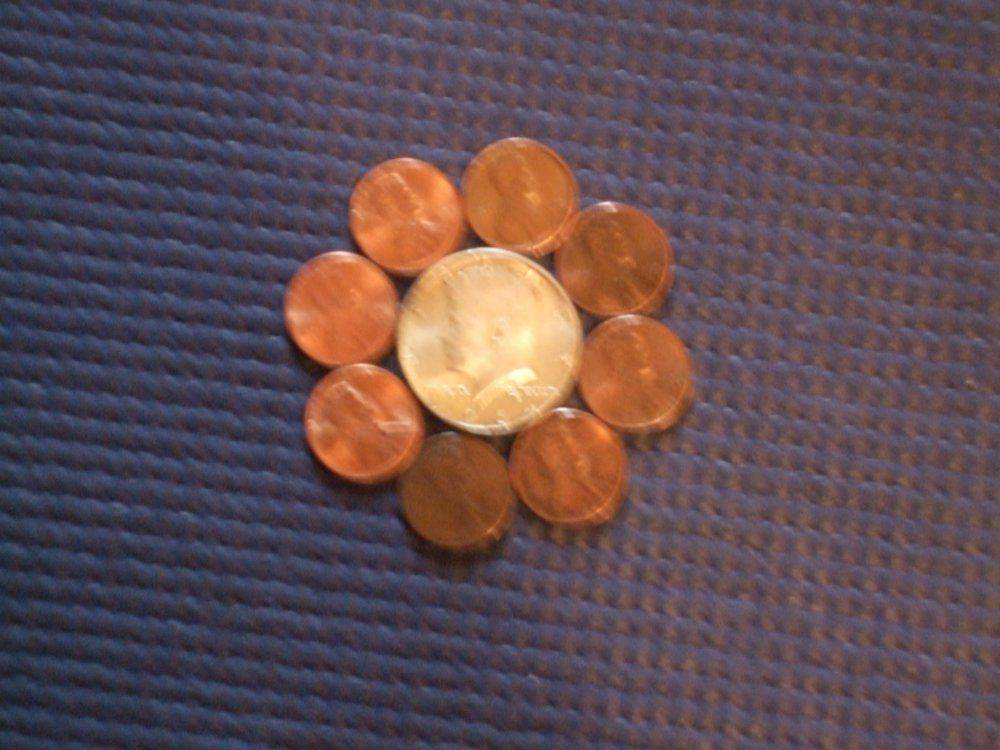# A simple trigonometry problem: Put eight coins around a central coin

Homework Helper
Gold Member
2020 Award
Summary:
A simple trigonometry problem: Put eight coins around a central coin. Compute the required ratio of the radii of the inner coin to the outer coin(s).
I have a simple trigonometry problem. I thought of making this as one of the math challenge problems, but it is almost too easy for that. ## \\ ## It is well known that 6 coins (circles) of equal size can be put around a center coin of the same radius, with the outer coins each touching two other outer coins plus the center coin. The problem is to compute with outer coin of radius "a" and inner coin of radius "b", what is the ratio of ## \frac{b}{a} ##, if there are 8 coins of radius ## a ## around the center coin of radius ## b ##? ## \\ ## Once you have solved for the answer, you might find it of interest that 8 U.S. pennies fit almost (but not quite) perfectly around a JFK 50 cent piece. If you google the sizes, you will see why.
## \\ ## Just for the fun of it, I welcome any high school level students to post an answer. ## \\ ## My post here is of a different nature than what I normally post= I'm hoping the section I picked is suitable for this post= @fresh_42 Hopefully this one is ok.

## Answers and Replies

Homework Helper
Gold Member
2020 Award
For coin sizes, see https://en.wikipedia.org/wiki/United_States_Mint_coin_sizes
## \\ ## And a hint to the above problem: It is a simple application of the law of cosines. ## \\ ## I will post the solution in a day or two if no one else posts it.## \\ ##
Edit: 30.6 mm/19.05 mm=1.606. As we see below, (post 3), the answer is 1.613 (approximately) so the JFK half dollar is just ever so slightly smaller than necessary to put the 8 pennies around it.

Last edited:
Homework Helper
Gold Member
2020 Award
And here is the solution: Let ## a ## be the radius of the smaller outer coins, and ## b ## the radius of the coin in the center. By the law of cosines ## C^2=A^2+B^2-2AB \cos{\theta} ##.
Here ## \theta=45^{\circ} ## because the circle gets divided in eight parts.
## C=2a ## , and ## A=B=a+b ##.
That makes ## 4a^2=2(a+b)^2-2(a+b)^2 \frac{\sqrt{2}}{2} ##.
This gives ## \sqrt{\frac{4}{2-\sqrt{2}}}-1=\frac{b}{a} \approx 1.613 ##.

Last edited:
Homework Helper
Gold Member
2020 AwardHere's the 8 pennies around the JFK half dollar. The last penny pushes the others out ever so slightly when it is put into place.

Last edited: